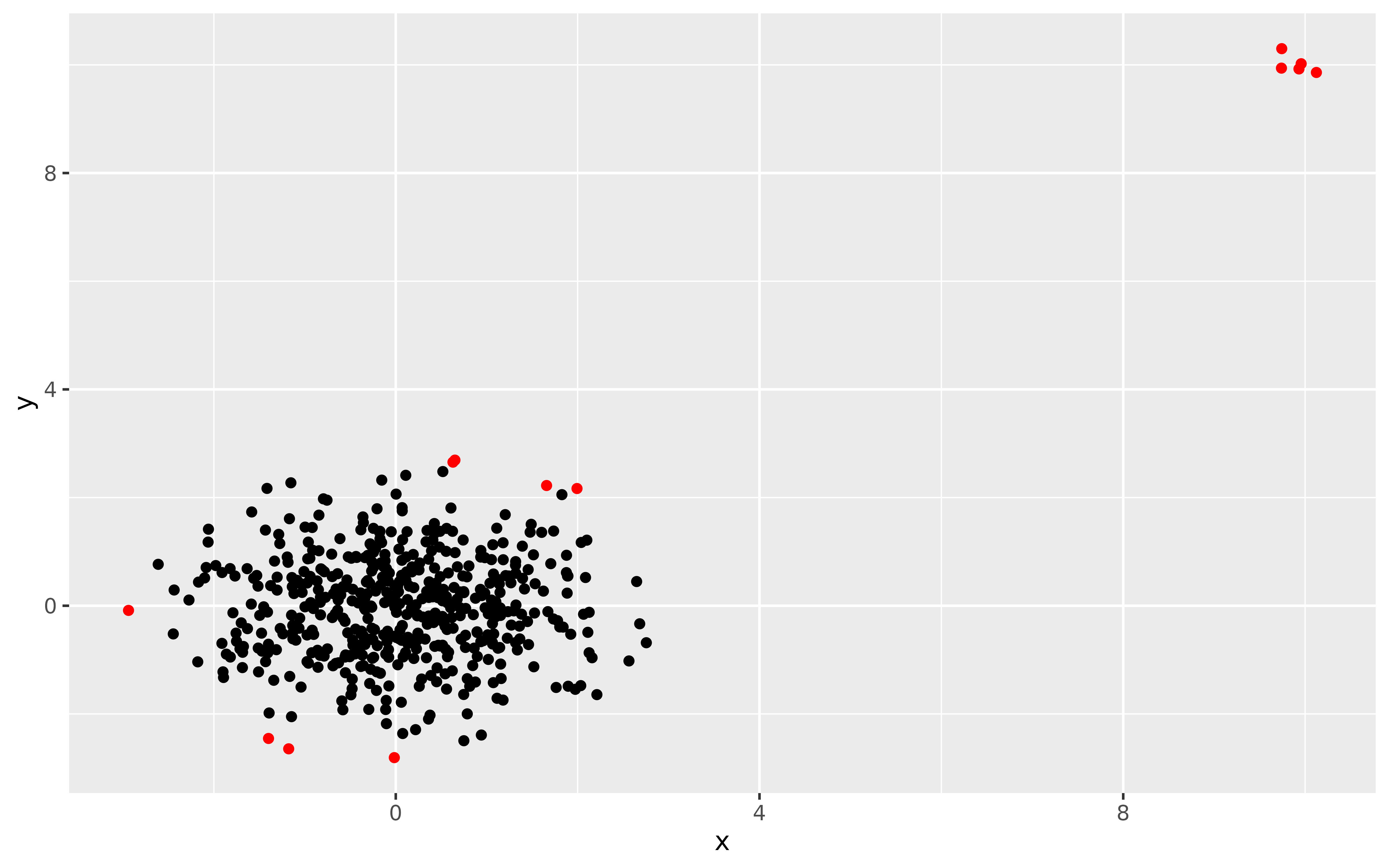Scatterplot of two columns from the data set with outliers highlighted.

# S3 method for lookoutliers
autoplot(object, columns = 1:2, ...)

## Arguments

object The output of the function lookout. Which columns of the original data to plot (specified as either numbers or strings) Other arguments currently ignored.

A ggplot object.

## Examples

X <- rbind(
data.frame(x = rnorm(500),
y = rnorm(500)),
data.frame(x = rnorm(5, mean = 10, sd = 0.2),
y = rnorm(5, mean = 10, sd = 0.2))
)
lo <- lookout(X)
autoplot(lo)